Kinetics

# 70 Chemical Reaction Rates

### Learning Objectives

By the end of this section, you will be able to:

• Define chemical reaction rate
• Derive rate expressions from the balanced equation for a given chemical reaction
• Calculate reaction rates from experimental data

A rate is a measure of how some property varies with time. Speed is a familiar rate that expresses the distance traveled by an object in a given amount of time. Wage is a rate that represents the amount of money earned by a person working for a given amount of time. Likewise, the rate of a chemical reaction is a measure of how much reactant is consumed, or how much product is produced, by the reaction in a given amount of time.

The rate of reaction is the change in the amount of a reactant or product per unit time. Reaction rates are therefore determined by measuring the time dependence of some property that can be related to reactant or product amounts. Rates of reactions that consume or produce gaseous substances, for example, are conveniently determined by measuring changes in volume or pressure. For reactions involving one or more colored substances, rates may be monitored via measurements of light absorption. For reactions involving aqueous electrolytes, rates may be measured via changes in a solution’s conductivity.

For reactants and products in solution, their relative amounts (concentrations) are conveniently used for purposes of expressing reaction rates. For example, the concentration of hydrogen peroxide, H2O2, in an aqueous solution changes slowly over time as it decomposes according to the equation: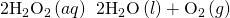The rate at which the hydrogen peroxide decomposes can be expressed in terms of the rate of change of its concentration, as shown here: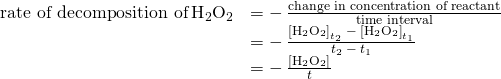This mathematical representation of the change in species concentration over time is the rate expression for the reaction. The brackets indicate molar concentrations, and the symbol delta (Δ) indicates “change in.” Thus,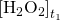represents the molar concentration of hydrogen peroxide at some time t1; likewise,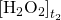represents the molar concentration of hydrogen peroxide at a later time t2; and Δ[H2O2] represents the change in molar concentration of hydrogen peroxide during the time interval Δt (that is, t2<!– no-selfclose –>t1). Since the reactant concentration decreases as the reaction proceeds, Δ[H2O2] is a negative quantity. Reaction rates are, by convention, positive quantities, and so this negative change in concentration is multiplied by −1. (Figure) provides an example of data collected during the decomposition of H2O2.

The rate of decomposition of H2O2 in an aqueous solution decreases as the concentration of H2O2 decreases.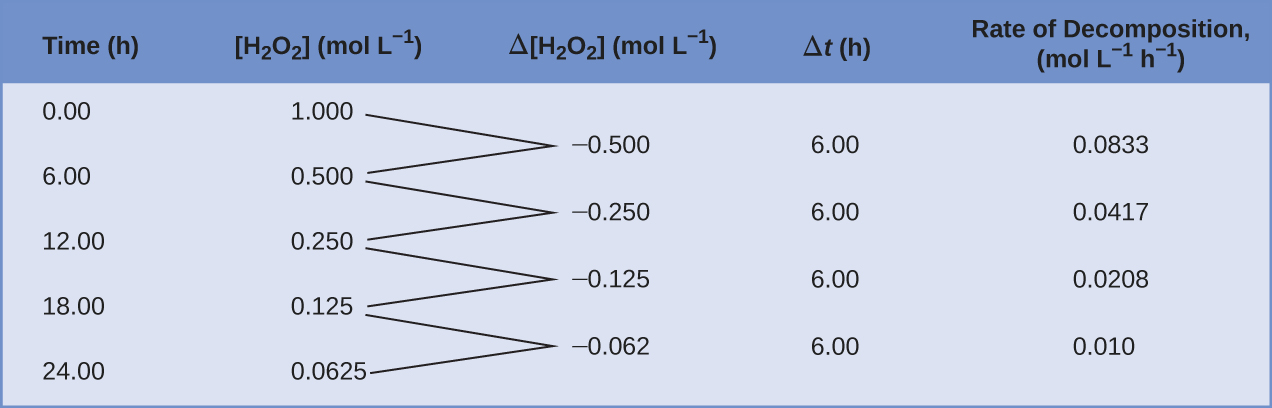To obtain the tabulated results for this decomposition, the concentration of hydrogen peroxide was measured every 6 hours over the course of a day at a constant temperature of 40 °C. Reaction rates were computed for each time interval by dividing the change in concentration by the corresponding time increment, as shown here for the first 6-hour period: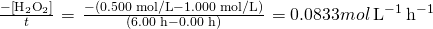Notice that the reaction rates vary with time, decreasing as the reaction proceeds. Results for the last 6-hour period yield a reaction rate of: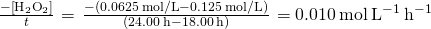This behavior indicates the reaction continually slows with time. Using the concentrations at the beginning and end of a time period over which the reaction rate is changing results in the calculation of an average rate for the reaction over this time interval. At any specific time, the rate at which a reaction is proceeding is known as its instantaneous rate. The instantaneous rate of a reaction at “time zero,” when the reaction commences, is its initial rate. Consider the analogy of a car slowing down as it approaches a stop sign. The vehicle’s initial rate—analogous to the beginning of a chemical reaction—would be the speedometer reading at the moment the driver begins pressing the brakes (t0). A few moments later, the instantaneous rate at a specific moment—call it t1—would be somewhat slower, as indicated by the speedometer reading at that point in time. As time passes, the instantaneous rate will continue to fall until it reaches zero, when the car (or reaction) stops. Unlike instantaneous speed, the car’s average speed is not indicated by the speedometer; but it can be calculated as the ratio of the distance traveled to the time required to bring the vehicle to a complete stop (Δt). Like the decelerating car, the average rate of a chemical reaction will fall somewhere between its initial and final rates.

The instantaneous rate of a reaction may be determined one of two ways. If experimental conditions permit the measurement of concentration changes over very short time intervals, then average rates computed as described earlier provide reasonably good approximations of instantaneous rates. Alternatively, a graphical procedure may be used that, in effect, yields the results that would be obtained if short time interval measurements were possible. In a plot of the concentration of hydrogen peroxide against time, the instantaneous rate of decomposition of H2O2 at any time t is given by the slope of a straight line that is tangent to the curve at that time ((Figure)). These tangent line slopes may be evaluated using calculus, but the procedure for doing so is beyond the scope of this chapter.

This graph shows a plot of concentration versus time for a 1.000 M solution of H2O2. The rate at any time is equal to the negative of the slope of a line tangent to the curve at that time. Tangents are shown at t = 0 h (“initial rate”) and at t = 12 h (“instantaneous rate” at 12 h).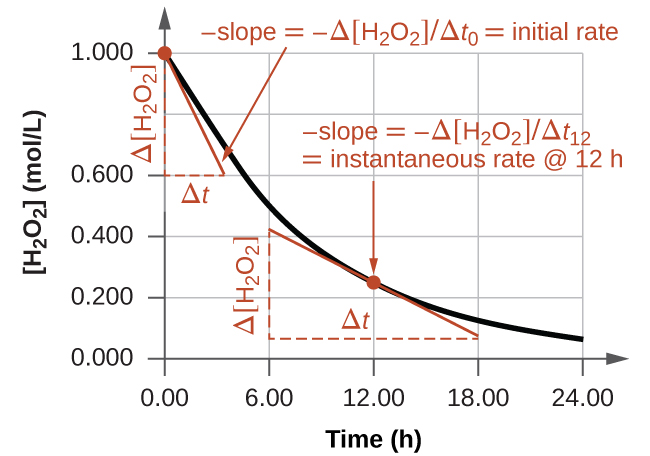Reaction Rates in Analysis: Test Strips for Urinalysis

Physicians often use disposable test strips to measure the amounts of various substances in a patient’s urine ((Figure)). These test strips contain various chemical reagents, embedded in small pads at various locations along the strip, which undergo changes in color upon exposure to sufficient concentrations of specific substances. The usage instructions for test strips often stress that proper read time is critical for optimal results. This emphasis on read time suggests that kinetic aspects of the chemical reactions occurring on the test strip are important considerations.

The test for urinary glucose relies on a two-step process represented by the chemical equations shown here: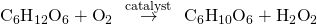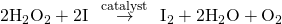The first equation depicts the oxidation of glucose in the urine to yield glucolactone and hydrogen peroxide. The hydrogen peroxide produced subsequently oxidizes colorless iodide ion to yield brown iodine, which may be visually detected. Some strips include an additional substance that reacts with iodine to produce a more distinct color change.

The two test reactions shown above are inherently very slow, but their rates are increased by special enzymes embedded in the test strip pad. This is an example of catalysis, a topic discussed later in this chapter. A typical glucose test strip for use with urine requires approximately 30 seconds for completion of the color-forming reactions. Reading the result too soon might lead one to conclude that the glucose concentration of the urine sample is lower than it actually is (a false-negative result). Waiting too long to assess the color change can lead to a false positive due to the slower (not catalyzed) oxidation of iodide ion by other substances found in urine.

Test strips are commonly used to detect the presence of specific substances in a person’s urine. Many test strips have several pads containing various reagents to permit the detection of multiple substances on a single strip. (credit: Iqbal Osman)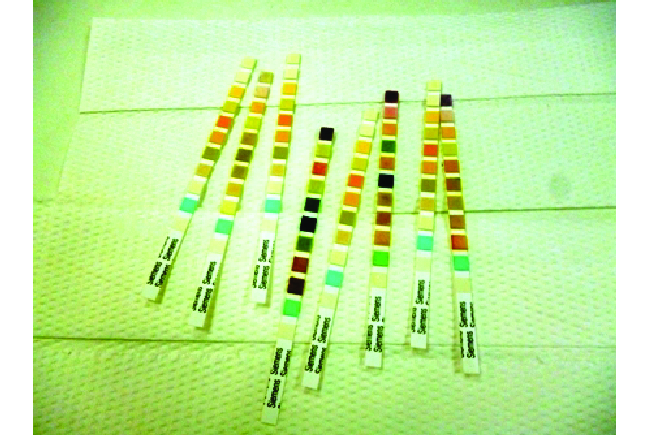### Relative Rates of Reaction

The rate of a reaction may be expressed as the change in concentration of any reactant or product. For any given reaction, these rate expressions are all related simply to one another according to the reaction stoichiometry. The rate of the general reaction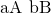can be expressed in terms of the decrease in the concentration of A or the increase in the concentration of B. These two rate expressions are related by the stoichiometry of the reaction: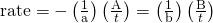the reaction represented by the following equation: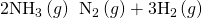The relation between the reaction rates expressed in terms of nitrogen production and ammonia consumption, for example, is: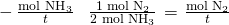This may be represented in an abbreviated format by omitting the units of the stoichiometric factor: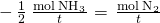Note that a negative sign has been included as a factor to account for the opposite signs of the two amount changes (the reactant amount is decreasing while the product amount is increasing). For homogeneous reactions, both the reactants and products are present in the same solution and thus occupy the same volume, so the molar amounts may be replaced with molar concentrations: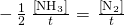Similarly, the rate of formation of H2 is three times the rate of formation of N2 because three moles of H2 are produced for each mole of N2 produced.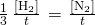(Figure) illustrates the change in concentrations over time for the decomposition of ammonia into nitrogen and hydrogen at 1100 °C. Slopes of the tangent lines at t = 500 s show that the instantaneous rates derived from all three species involved in the reaction are related by their stoichiometric factors. The rate of hydrogen production, for example, is observed to be three times greater than that for nitrogen production: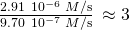Changes in concentrations of the reactant and products for the reaction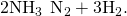The rates of change of the three concentrations are related by the reaction stoichiometry, as shown by the different slopes of the tangents at t = 500 s.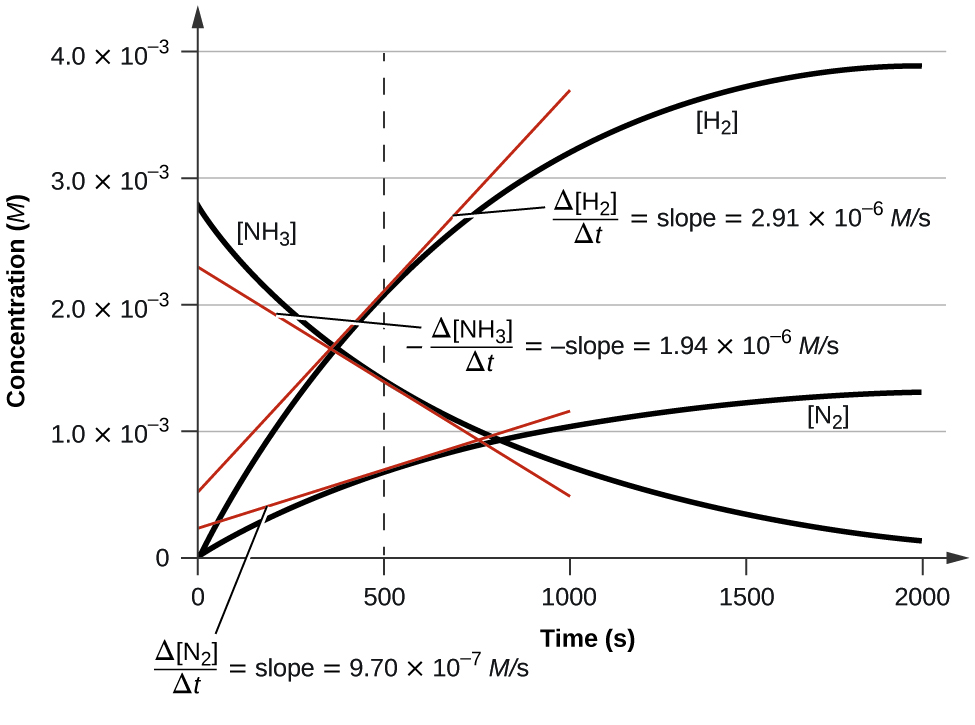Expressions for Relative Reaction Rates The first step in the production of nitric acid is the combustion of ammonia: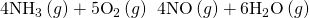Write the equations that relate the rates of consumption of the reactants and the rates of formation of the products.

Solution Considering the stoichiometry of this homogeneous reaction, the rates for the consumption of reactants and formation of products are: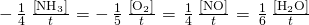Check Your Learning The rate of formation of Br2 is 6.010−6 mol/L/s in a reaction described by the following net ionic equation: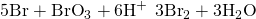Write the equations that relate the rates of consumption of the reactants and the rates of formation of the products.

Answer: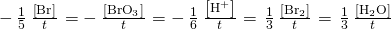Reaction Rate Expressions for Decomposition of H2O2 The graph in (Figure) shows the rate of the decomposition of H2O2 over time: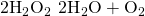Based on these data, the instantaneous rate of decomposition of H2O2 at t = 11.1 h is determined to be
3.2010−2 mol/L/h, that is: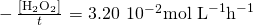What is the instantaneous rate of production of H2O and O2?

Solution The reaction stoichiometry shows that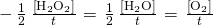Therefore: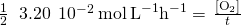and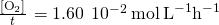Check Your Learning If the rate of decomposition of ammonia, NH3, at 1150 K is 2.1010−6 mol/L/s, what is the rate of production of nitrogen and hydrogen?

Answer:

1.0510−6 mol/L/s, N2 and 3.1510−6 mol/L/s, H2.

### Key Concepts and Summary

The rate of a reaction can be expressed either in terms of the decrease in the amount of a reactant or the increase in the amount of a product per unit time. Relations between different rate expressions for a given reaction are derived directly from the stoichiometric coefficients of the equation representing the reaction.

### Key Equations

•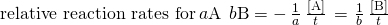### Chemistry End of Chapter Exercises

What is the difference between average rate, initial rate, and instantaneous rate?

The instantaneous rate is the rate of a reaction at any particular point in time, a period of time that is so short that the concentrations of reactants and products change by a negligible amount. The initial rate is the instantaneous rate of reaction as it starts (as product just begins to form). Average rate is the average of the instantaneous rates over a time period.

Ozone decomposes to oxygen according to the equation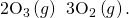Write the equation that relates the rate expressions for this reaction in terms of the disappearance of O3 and the formation of oxygen.

In the nuclear industry, chlorine trifluoride is used to prepare uranium hexafluoride, a volatile compound of uranium used in the separation of uranium isotopes. Chlorine trifluoride is prepared by the reaction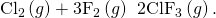Write the equation that relates the rate expressions for this reaction in terms of the disappearance of Cl2 and F2 and the formation of ClF3.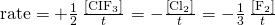A study of the rate of dimerization of C4H6 gave the data shown in the table: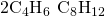Time (s) 0 1600 3200 4800 6200 [C4H6] (M) 1.0010−2 5.0410−3 3.3710−3 2.5310−3 2.0810−3

(a) Determine the average rate of dimerization between 0 s and 1600 s, and between 1600 s and 3200 s.

(b) Estimate the instantaneous rate of dimerization at 3200 s from a graph of time versus [C4H6]. What are the units of this rate?

(c) Determine the average rate of formation of C8H12 at 1600 s and the instantaneous rate of formation at 3200 s from the rates found in parts (a) and (b).

A study of the rate of the reaction represented as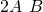gave the following data:

 Time (s) 0 5 10 15 20 25 35 [A] (M) 1 0.775 0.625 0.465 0.36 0.285 0.23

(a) Determine the average rate of disappearance of A between 0.0 s and 10.0 s, and between 10.0 s and 20.0 s.

(b) Estimate the instantaneous rate of disappearance of A at 15.0 s from a graph of time versus [A]. What are the units of this rate?

(c) Use the rates found in parts (a) and (b) to determine the average rate of formation of B between 0.00 s and 10.0 s, and the instantaneous rate of formation of B at 15.0 s.

(a) average rate, 0 − 10 s = 0.0375 mol L−1 s−1; average rate, 10 − 20 s = 0.0265 mol L−1 s−1; (b) instantaneous rate, 15 s = 0.023 mol L−1 s−1; (c) average rate for B formation = 0.0188 mol L−1 s−1; instantaneous rate for B formation = 0.012 mol L−1 s−1

Consider the following reaction in aqueous solution: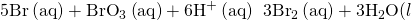If the rate of disappearance of Br(aq) at a particular moment during the reaction is 3.510−4 mol L−1s−1, what is the rate of appearance of Br2(aq) at that moment?

### Glossary

average rate
rate of a chemical reaction computed as the ratio of a measured change in amount or concentration of substance to the time interval over which the change occurred
initial rate
instantaneous rate of a chemical reaction at t = 0 s (immediately after the reaction has begun)
instantaneous rate
rate of a chemical reaction at any instant in time, determined by the slope of the line tangential to a graph of concentration as a function of time
rate of reaction
measure of the speed at which a chemical reaction takes place
rate expression
mathematical representation defining reaction rate as change in amount, concentration, or pressure of reactant or product species per unit time

## LicenseChemistry 2e by OSCRiceUniversity is licensed under a Creative Commons Attribution 4.0 International License, except where otherwise noted.